# HSPT Quantitative : How to make geometric comparisons

## Example Questions

### Example Question #11 : Geometric Comparison

Examine (a), (b), and (c) to find the best answer:

a) the diameter of a circle

b) twice the radius of a circle

c) the circumference of a circle divided by(a), (b), and (c) are all not equivalent

(a) is equivalent to (b) but not (c)

(a) is equivalent to (c) but not (b)

(a), (b), and (c) are all equivalent

(a), (b), and (c) are all equivalent

Explanation:

Diameter is found using this formula, which shows that it is twice the radius: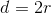Circumference is found using this formula: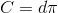Manipulate this to find that diameter is also circumference divided by: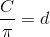Therefore, all of these expressions are the same.

### Example Question #12 : How To Make Geometric Comparisons

Examine (a), (b), and (c) to find the best answer:

a) perimeter of a square with an area ofb) perimeter of a square with an area ofc) perimeter of a square with a side length of(a) is equal to (c)

(c) is two times (b)

(c) is two times (a)

(b) is equal to (c)

(c) is two times (b)

Explanation:

If you have the area of a square, you can find the side length by finding the square root. Then multiply by four to find the perimeter:

a)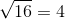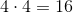b)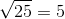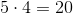If you are given the side length, simply multiply by: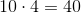### Example Question #13 : How To Make Geometric Comparisons

Examine (a), (b), and (c) to find the best answer:

(a) area of an equilateral triangle

(b) area of an isoceles triangle

(c) area of a right triangle

(c) is the greatest

(b) is the greatest

It is impossible to say which is greatest.

(a) is the greatest

It is impossible to say which is greatest.

Explanation:

The shape of a triangle does not affect its size. Without knowing any dimensions, we cannot determine how large any of the areas are.

### Example Question #14 : How To Make Geometric Comparisons

Circle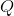has a radius ofinches, a circumference ofinches, and an area ofinches squared.

Now imagine three other circles:

a) Circlehas a radius of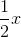b) Circlehas a circumference of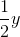c) Circlehas an area of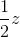Circleis equivalent to Circlebut notCircles, andare all nonequivalent

Circleis equivalent to Circlebut notCircles, andare all equivalent

Circleis equivalent to Circlebut notExplanation:

Radius and circumference are related to each other by a direct proportion (). This means that if you cut one in half, you cut the other in half. For this reason, circlesandare both related toby the same proportion and are thus equivalent.

Area is related to radius exponentially (). Cutting the area in half does not cut the radius in half. Circletherefore cannot be equivalent to.

To test this out, try substituting some numbers in for, and.

### Example Question #11 : How To Make Geometric Comparisons

Examine (a), (b), and (c) to find the best answer:

a) volume of a cube with side length ofinches

b) area of a square with a perimeter ofinches

c) perimeter of a square with a side length ofinches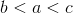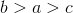Impossible to compare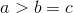Impossible to compare

Explanation:

Voume would be measured in inches cubed, area in inches squared, and perimeter in inches. These are different types of measurements and cannot be compared.

### Example Question #16 : How To Make Geometric Comparisons

Examine (a), (b), and (c) to find the best answer:

a) area of a circle with a circumference of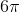b) area of a circle with a radius ofc) area of a circle with a diameter of(b) is equal to (c) but not (a)

(a), (b), and (c) are all equal

(a) is equal to (c) but not (b)

(a), (b), and (c) are all unequal

(a), (b), and (c) are all equal

Explanation:

The diameter of a circle is twice its radius. Since the diameter in (c) is twice the radius in (b), these two are equal.

The circumference is the diameter multiplied by. Since the circumfrence in (a) is the diameter in (c) multiplied by, this is also equal.

### Example Question #11 : How To Make Geometric Comparisons

Examine (a), (b), and (c) to find the best answer:

a) perimeter of a square with a side length ofb) circumference of a circle with a diameter ofc) perimeter of an equilateral triangle with a side length of(a) is greater than (b) and (c).

(b) is equal to (c).

(a) is equal to (c).

(b) is greater than (a) and (c).

(a) is equal to (c).

Explanation:

Find perimeter by adding up the lengths of the sides of the shape. Find circumference by multiplying the diameter by:

a) perimeter of a square with a side length ofb) circumference of a circle with a diameter of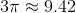c) perimeter of an equilateral triangle with a side length of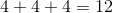Therefore (a) and (c) are equal, and they are greater than (b).

### Example Question #18 : How To Make Geometric Comparisons

Examine (a), (b), and (c) to find the best answer:

a) perimeter of an equilateral triangle with a side length ofb) perimeter of a square with an side length ofc) circumference of a circle with a diameter of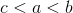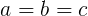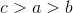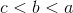Explanation:

Find the perimeter from side length of an equilateral triangle by multiplying by three:

a)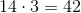Find perimeter from side length of a square by multiplying by four:

b)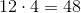Find circumference from diameter of a circle by multiplying by pi:

c)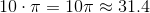It is now evident that (c) is smaller than (a), which is smaller than (b)

### Example Question #19 : How To Make Geometric Comparisons

Examine (a), (b), and (c) to find the best answer:

a) the sum of the areas of a square and a triangle

b) the sum of the areas of two squares

c) the sum of the areas of two trianglesNot enough information to know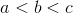Not enough information to know

Explanation:

We are not given any information about the sizes of the shapes. There could be two tiny squares, one huge triangle, we're not sure. Without any dimensions, we cannot say which area is bigger.

### Example Question #20 : How To Make Geometric Comparisons

Compare the values of (a), (b), and (c):

(a) The area of a square with a perimeter of(b) The area of a square with a side length of(c) The area of a square with a perimeter of(a), (b), and (c) are all unequal

(a), (b), and (c) are all equal

(b) is equal to (c), but not (a)

(a) is equal to (b), but not (c)

(a) is equal to (b), but not (c)

Explanation:

Find the areas of all the squares. To find an area from a perimeter, divide the perimeter byto find the side length, then square the side length.

(a)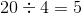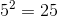(b)(c)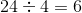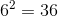(a) is equal to (b), but not equal to (c).